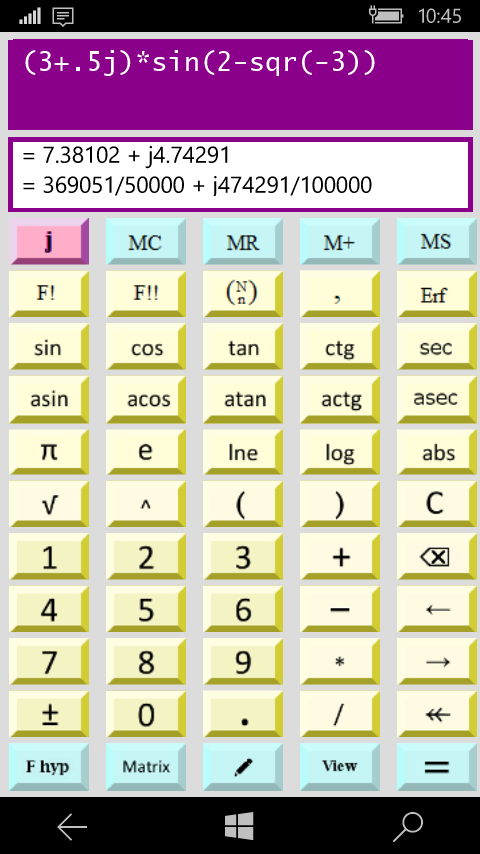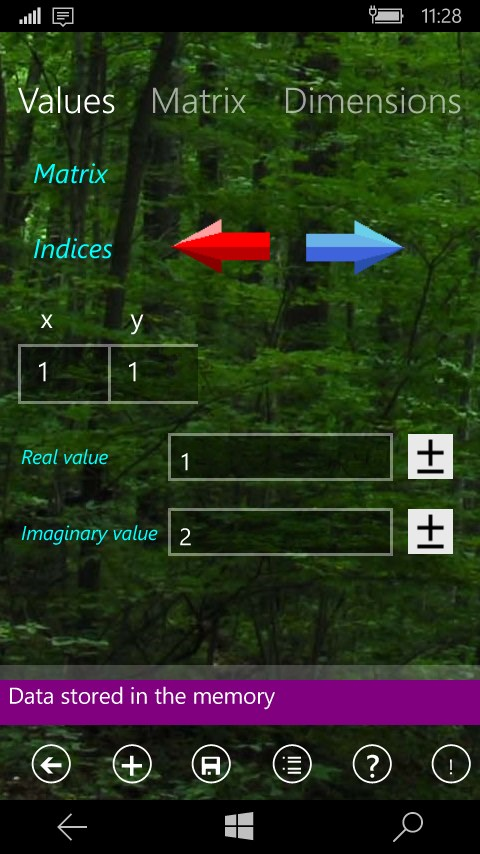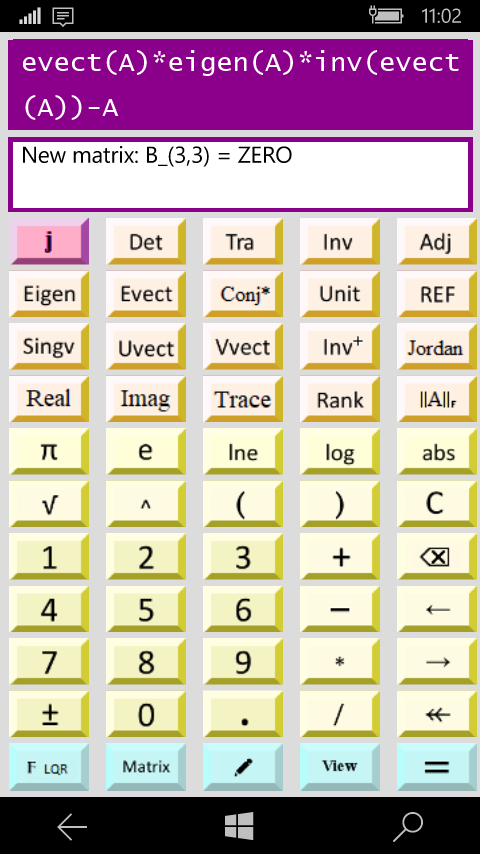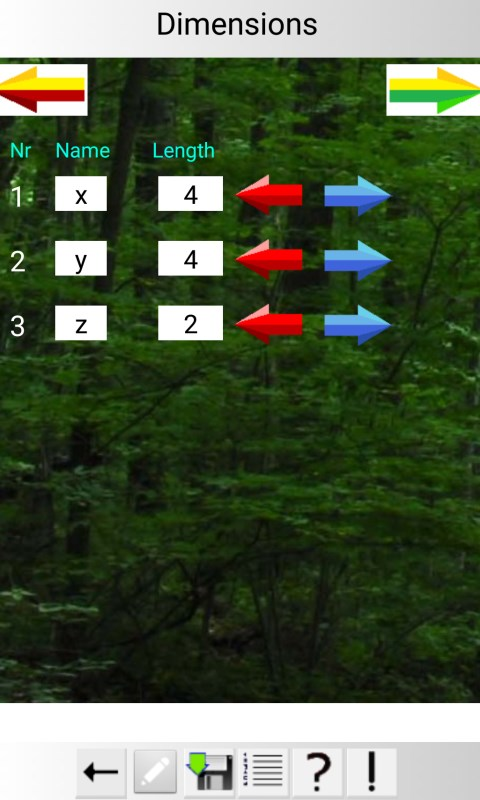Supported Devices
PC,Mobile,Hub
Updated
May 15,2019
Size
21.89 MB
Age
Everyone
Release Date
Description
The possible operations are the standard of mathematics and the following matrix operations:

* = product matrix
/ = division of two matrices, or product of the inverse matrix
^ = power of a matrix (only with integers)
+ = sum matrix
- = difference matrix
Det = Determinant
Tra = matrix transpose
Inv = matrix inverse
tr(A) = trace of matrix A
Unit = matrix unit
Rank = matrix rank
Erf = error function erf
REF = matrix in Row Echelon Form (system solution)
The following matrix operations are operative only with the Pro version:
Inv+ = Moore - Penrose pseudo inverse
Eigen = matrix eigenvalues
Evect = matrix eigenvectors
Vsing = matrix singular values S
Uvect = left vector singular matrix U
Vvect = right vector singular matrix V
Dsum = matrix direct sum
Outer = outer product
L(L*L') = Lower triangolar matrix L so that A = L*L'
Q(Q*R) = Left matrix Q so that A = Q*R
R(Q*R) = Wright matrix R so thar A = Q*R
Jordan = Jordan matrix J
||A|| = Frobenius norm
e^A = exponential of matrix A
√ A = square root matrix